﻿ A quick and effective multivariate statistical strategy for imaging mass spectrometry
 Chinese Chemical Letters2014, Vol.25Issue (10):1331-1335PDF
A quick and effective multivariate statistical strategy for imaging mass spectrometry
Fei Tanga, Yi Chena, Tie-Gang Lib, Jiu-Ming Heb, Zeper Ablizb, Gang Huangc, Xiao-Hao Wangaa State Key Laboratory of Precision Measurement Technology and Instruments, Department of Precision Instrument, Tsinghua University, Beijing 100084, China;
b State Key Laboratory of Bioactive Substance and Function of Natural Medicines, Institute of Materia Medica, Chinese Academy of Medical Sciences and Peking Union Medical College, Beijing 100050, China;
c National Key Laboratory of Human Factors Engineering, China Astronaut Research and Training Center, Beijing 100094, China
Abstract: A new multivariate statistical strategy for analyzing large datasets that are produced by imaging mass spectrometry (IMS) techniques is reported. The strategy divides the whole datacube of the sample into several subsets and analyses them one by one to obtain the results. Instead of analyzing the whole datacube at one time, the strategy makes the analysis easier and decreases the computation time greatly. In this report, the IMS data are produced by the air flow-assisted ionization IMS (AFAI-IMS). The strategy can be used in combination with most multivariate statistical analysis methods. In this paper, the strategy was combined with the principal component analysis (PCA) and partial least square analysis (PLS). It was proven to be effective by analyzing the handwriting sample. By using the strategy, the m/z corresponding to the specific lipids in rat brain tissue were distinguished successfully. Moreover the analysis time grew linearly instead of exponentially as the size of sample increased. The strategy developed in this study has enormous potential for searching for the m/z of potential biomarkers quickly and effectively.
Key words: Imaging mass spectrometry     Multivariate statistical analysis     Potential biomarkers     Quick and effective
1. Introduction

In recent years,imaging mass spectrometry (IMS),a new field in mass spectrometry research,has developed rapidly. IMS images contain information on hundreds or even thousands of different molecules in the samples,and both molecular and spatial information can be obtained through a single sample analysis [1, 2]. IMS can be used to track the spatial distribution of specific molecules in diseased and normal tissue. It plays an important role in various applications,such as medical diagnostics,physiological and pathological investigation,and biomarker assays [3, 4, 5].

Many IMS technologies have been developed,including matrixassisted laser desorption ionization IMS (MALDI-IMS) [6, 7], desorption electrospray ionization IMS (DESI-IMS) ,and air flow-assisted ionization IMS (AFAI-IMS) ,among others. Since the development of IMS,its pixel resolution and mass resolution have continuously improved,resulting in a large amount of mass spectrometry data. As a result,extracting,classifying,and sorting the effective information from the original mass spectrum data has become a focus of current research [10, 11]. Due to the large size of mass spectrum data,it is difficult to determine particular m/z of biomarkers through manual screening and checking. Statistical analysis,data dimensionality reduction and extraction,image processing,and analysis are necessary before using IMS data for various applications.

Multivariate statistical analysis is a suitable method to achieve this purpose [12, 13, 14, 15, 16]. Multivariate statistics is a general statistical method used to process more than one variable. There are many different models of it,such as principal component analysis (PCA) [17, 18],hierarchical cluster analysis (HCA)  and partial least square discriminate analysis (PLS-DA) . Good results have been obtained when applying these methods to IMS data processing.

Most of the current methods for multivariate statistical analysis are used to analyze the complete mass spectrometry datacube of the entire sample at one time. However,this is a difficult and time-consuming strategy because the whole datacube is too large [21, 22].

In this paper,we propose a new strategy that can be used in combination with most of the multivariate statistical analysis methods. When applying this strategy,the whole mass spectrometry datacube of the sample was first divided into several subsets. Each of the subsets then was analyzed one by one by a particular multivariate statistical method (e.g. PCA) to get the initial results. The final multivariate statistical analysis result of the whole datacube was obtained by adding the entire initial results together. In doing so,the specific m/z reflecting the composition distribution of sample can be effectively determined.

PCA and PLS algorithms from MATLAB have been well developed and hence can be applied in most IMS technologies. These two methods were adopted in this paper as multivariate statistical methods to analyze the treated data. The strategy used in combination with these two multivariate statistical methods was validated by analyzing handwriting samples. The analysis results clearly show that the m/z of the two components in the sample was correctly extracted. The strategy was preliminarily applied for samples of rat brain tissue slices,and the m/z corresponding to tissue slice contours and particular lipids were determined. More importantly,the strategy reduced the analysis time drastically. By using this strategy,the analysis time grew linearly instead of exponentially as the amount of data to be analyzed increased.

This strategy has enormous potential for searching for the m/z of potential biomarkers both quickly and effectively. It can facilitate the research on the large and whole-body IMS technology,clinical applications,cancer diagnosis,etc. It extends the application fields of IMS technology. 2. Experimental

2.1. Instruments The IMS technology used in this paper was based on the AFAIIMS. The experiments were performed using the QTRAP 5500 and QSTAR Elite QTOF mass spectrometers (AB SCIEX Foster City,CA, USA). The ion sources of the mass spectrometers were replaced with the AFAI source,which includes an ESI spray needle,an ion transport tube,a mass spectrometer interface,and a pump .

The ESI spray gas was N2and had a flow rate of 2 L/min. The voltage of the ESI needle was 5000 V,and the spray solution was the mixture of methanol and water (4:1,v/v) with 0.1% formic acid . The spray solution was delivered to the needle with an Agilent LC pump with a liquid flow rate of 10 mL/min. The assisting air flow rate of the AFAI source was 40 L/min .

The data processing program was implemented using MATLAB, which integrated the imaging and multivariate analysis functions. The PCA and PLS algorithms were included in the program, including the import of mass spectrometry data,data reconstruction,and multivariate statistical analysis. The results of PCA and PLS analysis were given by scatter plots,with each data point representing one m/z. The value of the m/z corresponding to a specific point can be obtained by selecting it directly from the program. 2.2. Sample preparation As shown in Fig. 1,sample (a) was used to validate the strategy and to compare the results of the PCA and PLS analysis. The letters ‘‘M’’ and ‘‘S’’ were written on a glass slide with either red or blue ink. The main components of the two inks were Rhodamine B (m/ z = 443.2) and Basic Blue 7 (m/z = 478.4). The size of the glass slide was 150 mm × 50 mm,and analysis area was 100 mm × 30 mm, as shown in Fig. 1a. The range of the m/z was 100-500 when the sample (a) was analyzed.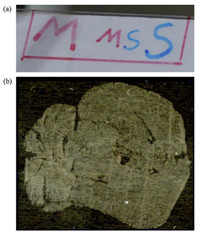Download: larger image Fig. 1. Samples to be analyzed: (a) sample with character written in red and blue inks. (b) Rat brain tissue slices.

Sample (b) was a rat brain tissue slice that contained some lipids,for which the m/z of the potential biomarkers was unknown. The rat was euthanized by ether overdose,frozen entirely in dry ice/isopentane,and then prepared for slicing. The Leica CM3600 cryomacrotome was used to obtain 20 mm rat brain tissue slices. The sample size was 15 mm × 14 mm and the slice was pasted on the glass slide. The range of the m/z was 500-999 when the sample (b) was analyzed. 2.3. The strategy In this paper,the mass spectrometer collected data at regular intervals. The distance between two adjacent sample points analyzed by the mass spectrometer was 200 mm. In other words, the spatial resolution of AFAI-IMS was 200 mm. One mass spectrometry data corresponded to one sample point and contained the ion intensity of the m/z. The data of the whole sample formed a three-dimensional datacube (X,Y,m/z),as shown in Fig. 2. Each element of the datacube was the ion intensity corresponding to one particular m/z at one particular position (X, Y).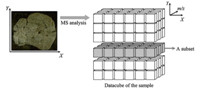Download: larger image Fig. 2. The datacube of the sample. It was the original data to be analyzed by the multivariate statistical strategy. The (X,Y) was the length and the width of the sample. A subset of the datacube was a dataset which had same X or Y.

To make the analysis easier and reduce the analysis time,the strategy reported in this paper did not analyze the whole datacube of the sample at one time. Instead,the datacube was divided into several subsets at first,as shown in Fig. 2. In this study,a subset was simply defined as a dataset with the same X or the same Y. The data with the same X or Y corresponded to the sample points that were in the same column or row of the sample. So,one subset corresponded to one sample row or column.

All of the subsets were analyzed one by one by the specific multivariate statistical methods (e.g. PCA) to get the initial results. Each initial result was composed of the scores of the whole m/z on multivariate statistical methods.

The subsets were of equal value and importance. So,the final multivariate statistical analysis result of the whole datacube was obtained by adding the scores of the same m/z in different initial results together. In this paper,all the subsets were defined as a dataset with the same Y-that was,one sample row’s data formed a subset.

Additionally,the subset was a dataset of the same X or same Y: it was a two-dimensional data matrix whose rows corresponded to different Y or X and columns corresponded to different m/z, respectively. 3. Results and discussion 3.1. Strategy validation and comparison The size of sample (a) was 100 mm × 30 mm,and for a 200 mm spatial resolution,the sample points which were analyzed by MS formed a 500 × 150 matrix. So,there were 150 subsets to be analyzed one by one. Each subset was analyzed by PCA to get the initial results. As shown in Fig. 3a,the final multivariate statistical analysis result was obtained by adding the entire series of initial results together. Each point in Fig. 3a-c represents one m/z. Generally,the point that had the highest absolute score values in the first principal component (PC1) and the second principal component (PC2) was the most special point in the sample,and the corresponding m/z was the characteristic m/z of the sample. For practical applications,several points that had absolute values with the highest scores shall be selected as alternatives. In accordance with the actual conditions,one could identify the characteristic m/ z of the components of the sample more accurately. Similarly,the analysis results can be derived using PLS to analyze each subset and adding the entire initial results together. The analysis results that we obtained are shown in Fig. 3b. To compare the analysis effects of the PCA and PLS,the analysis results were rotated to make observations easier. The contrasting result that we obtained is shown in Fig. 3c.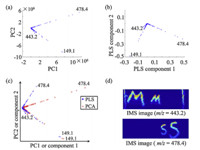Download: larger image Fig. 3. Multivariate statistical analysis result of the strategy on sample (a). (a) Result of the strategy based on PCA. (b) Result of the strategy based on PLS. (c) Rotated contrast results of strategy based on PCA and PLS. (d) IMS images of Sample (a) at m/z = 478.4 and m/z = 443.2.

The known m/z of the main components of the two kinds of inks were 443.2 (red) and 478.4 (blue). The m/z = 149.1 obtained from Fig. 3a and b could be considered as the background,and the other two m/z with higher scores were exactly 478.4 and 443.2,which were consistent with the known m/z. The IMS image of m/z = 443.2 and m/z = 478.4 also supports this conclusion,as shown in Fig. 3d. As shown clearly in Fig. 3c,the separation angles of m/z = 478.4 and m/z = 149.1 obtained from the results of the PLS was greater, and the separation angles of m/z = 443.1 and m/z = 149.1 were also slightly larger than the PCA. These results indicate that the PLS could better distinguish the background and sample,thus giving better analysis results. Therefore,the PLS method was better for determining the m/z that correspond to possible potential biomarkers. 3.2. Analysis of rat brain tissue slices After proving the strategy was effective,we applied it to analyze a more complex sample. The sample of rat brain tissue slices was 15 mm × 14 mm,and for a 200 mm spatial resolution,the sample points which were analyzed by MS formed a 75 × 70 matrix. The strategy divided the datacube of the sample (b) into 70 subsets. All of the subsets were subjected to the PCA or PLS method one by one to get the initial results. The final result was obtained by adding the initial results together. As shown in Fig. 4,the m/z representing the components of the tissue was identified.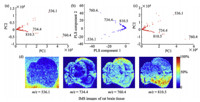Download: larger image Fig. 4.The analysis results of strategy based on (a) PCA and (b) PLS of rat brain tissue slices. (c) The analysis results of the previous method based on PCA. (d) IMS images of the special m/z.

The special m/z could be obtained by selecting the m/z points with higher scores in Fig. 4a-c. The m/z of the potential biomarkers may be: m/z = 536.1,734.4,760.4,and 810.5. IMS Imaging results of the above m/z with the AFAI-IMS program were shown in Fig. 4d.

As shown in Fig. 4d,the ion intensity of the sample components corresponding to the m/z = 536.1 was higher in the area outside the rat brain tissue slices,and thus,it must represent the background. The m/z = 734.4,760.4,and 810.5 clearly reflect the area where the rat brain tissue slices were located,as well as the distribution of the corresponding substances in the tissue slices. Therefore,the special m/z required could be successfully extracted by the strategy. Currently,only the fact that these substances were lipids was understood,and the structures were not discussed in this paper.

The whole datacube of the rat brain tissue was analyzed by PCA at one time and the result was shown in Fig. 4c. Comparing Fig. 4a and c,the result of the outlined strategy was almost identical with the result obtained by analyzing the whole datacube at one time. So,the strategy was proven to be effective. 3.3. Analysis time of the strategy Since the development of IMS,its pixel resolution and mass resolution have been continuously improved,resulting in a large amount of mass spectrometry data. The previous method normally conducted the multivariate statistical analysis for large datacube at one time,resulting in long analysis times. The strategy reported in this paper divided the large datacube into several subsets,and then conducted the multivariate statistical analysis for each subset.

The strategy reduced the analysis time greatly. To prove this,we compared the previous method and the strategy in this experiment. The two methods were both based on PCA. Different parts of the sample (b) were used for experimentation. For example,a part of sample (b) whose size was 15 mm × 0.2 mm was analyzed as a minimum sample,and the whole sample (b) whose size was 15 mm × 14 mm was analyzed as a maximum sample. The minimum sample was not divided,as it corresponded to one subset in the experiment,and all of the samples whose sizes were different had the same definition of the subsets. For example,the minimum sample had one subset,the sample whose size was 15 mm × 0.4 mm was divided into two subsets,and the whole sample (b) whose size was 15 mm × 14 mm divided into 70 subsets. The analysis times of the previous method and the strategy that we obtained are shown in Fig. 5.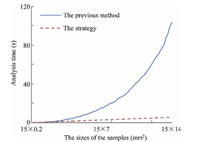Download: larger image Fig. 5.The analysis times needed to analyze the different size of the sample on previous method and the strategy reported in this paper.

As shown in Fig. 5,as the size of the data increased,the analysis time of the previous method increased exponentially,while the analysis time of the proposed strategy increased only linearly. If the analysis was conducted for each subset separately (the strategy),the total analysis time was only nT,where n is the number of the subsets and T is the time required for the analysis on one subset,but if the multivariate statistical analysis was conducted for all the mass spectrometry data simultaneously (the previous method),the time consumed was X n (X may be a functional expression). For example,to analyze the minimum sample whose size was 15 mm × 0.2 mm,the analysis time was 0.078 s according to the experiment result. As the size of the sample increased to 15 mm × 6 mm,it was divided into 30 subsets. Theoretically,the analysis time of the strategy was 2.34 s (30 s × 0.078 s),and it matched with the value obtained by experiment (2.36 s). And according to the experiment,the analysis time of the previous method was 10.55 s.

The strategy we describe here provides a new way to analyze the large datacube of the sample: the multivariate statistical analysis was conducted for each subset of datacube one by one. The multivariate statistical analysis result for all m/z in each subset was obtained at this time. Then the results for the same m/z in different subsets were added together as the final result. 4. Conclusion Based on the results of experiments,the strategy was proven to be effective. The substances withspecialm/zextractedfromthe rat brain tissue slices are considered to be lipids,and their structures will be studied further. Through the research on the multivariate statistical analysis time,we found that the analysis time of the previous method increased exponentially as the amount of data increased,and such a high time complexity makes the multivariate statistical analysis of large datacubes prohibitive. Thus,this strategy was of practical significance for saving time and making analysis easier.

Using the strategy proposed in this paper,the special m/z can be extracted and the difficulty and time needed for data analysis can be greatly reduced. Therefore,the requirement of running the data processing program can be reduced,and the required computing power of the computer is reduced. These findings are of great significance for the development of portable IMS devices. The application of this strategy has the significant ability to identify potential biomarkers.

Acknowledgments This work is financially supported by the National Instrumentation Programmme (Nos. 2011YQ17006702 and 2011YQ14015010), the National Natural Science Foundation of China (Nos. 81102413 and 21175121),and Fundamental Research Program of Shenzhen (No. JC201005280634A)
References
  L.A. McDonnell, R.M.A. Heeren, Imaging mass spectrometry, Mass Spectrom. Rev. 26 (2007) 606-643.  S. Shimma, M. Setou, Review of imaging mass spectrometry, J. Mass Spectrom. Soc. Jpn. 53 (2005) 230-238.  L.S. Eberlin, A.L. Dill, A.J. Golby, et al., Discrimination of human astrocytoma subtypes by lipid analysis using desorption electrospray ionization imaging mass spectrometry, Angew. Chem. Int. Ed. Engl. 49 (2010) 5953-5956.  P.H. Pevsner, J. Melamed, T. Remsen, et al., Mass spectrometry MALDI imaging of colon cancer biomarkers: a new diagnostic paradigm, Biomakers Med. 3 (2009) 55-69.  E.H. Seeley, R.M. Caprioli, MALDI imaging mass spectrometry of human tissue: method challenges and clinical perspectives, Trends Biotechnol. 29 (2011) 136-143.  S.A. Schwartz, M.L. Reyzer, R.M. Caprioli, Direct tissue analysis using matrixassisted laser desorption/ionization mass spectrometry: practical aspects of sample preparation, J. Mass Spectrom. 38 (2003) 699-708.  R.M. Caprioli, T.B. Farmer, J. Gile, Molecular imaging of biological samples: localization of peptides and proteins using MALDI-TOF MS, Anal. Chem. 23 (1997) 4751-4760.  R.I. Demian, M.W. Justin, Q. Song, R.G. Cooks, Development of capabilities for imaging mass spectrometry under ambient conditions with desorption electrospray ionization (DESI), Int. J. Mass Spectrom. 259 (2007) 8-15.  Z.G. Luo, J.M. He, Y.J. Chen, et al., Air flow-assisted ionization imaging mass spectrometry method for easy whole-body molecular imaging under ambient conditions, Anal. Chem. 85 (2013) 2977-2982.  J.M. Fonville, C. Carter, O. Cloarec, et al., Robust data processing and normalization strategy for MALDI mass spectrometric imaging, Anal. Chem. 84 (2012) 1310-1319.  D. Trede, J.H. Kobarg, J. Oetjen, et al., On the importance of mathematical methods for analysis of MALDI-imaging mass spectrometry data, J. Integr. Bioinform. 9 (2012) 189.  E.A. Jones, A. Remoortere, R.J.M. Zeijl, et al., Multiple statistical analysis techniques corroborate intratumor heterogeneity in imaging mass spectrometry datasets of myxofibrosarcoma, PLoS ONE 6 (2011) 1-14.  W. Reindl, B.P. Bowen, M.A. Balamotis, J.E. Greenc, T.R. Northen, Multivariate analysis of a 3D mass spectral image for examining tissue heterogeneity, Integr. Biol. 3 (2011) 460-467.  B.J. Tylera, G. Rayala, D.G. Castner, Multivariate analysis strategies for processing ToF-SIMS images of biomaterials, Biomaterials 28 (2007) 2412-2423.  V.S. Smentkowski, S.G. Ostrowski, F. Kollmer, et al., Multivariate statistical analysis of non-mass-selected ToF-SIMS data, Surf. Interface Anal. 40 (2008) 1176-1182.  A.L. Dill, L.S. Eberlin, C. Zheng, et al., Multivariate statistical differentiation of renal cell carcinomas based on lipidomic analysis by ambient ionization imaging mass spectrometry, Anal. Bioanal. Chem. 398 (2010) 2969-2978.  Z.Z. Pan, H.W. Gu, N. Talaty, et al., Principal component analysis of urine metabolites detected by NMR and DESI-MS in patients with inborn errors of metabolism, Anal. Bioanal. Chem. 387 (2007) 539-549.  H.W. Gu, Z.Z. Pan, B.W. Xi, et al., Principal component directed partial least squares analysis for combining NMR and MS data in metabolomics: application to the detection of breast cancer, Anal. Chim. Acta 686 (2011) 57-63.  D. Bonnel, R. Longuespee, J. Franck, et al., Multivariate analyses for biomarkers hunting and validation through on-tissue bottom-up or in-source decay in MALDI-MSI: application to prostate cancer, Anal. Bioanal. Chem. 401 (2011) 149-165.  V. Pirro, L.S. Eberlin, P. Oliveric, R.G. Cooks, Interactive hyperspectral approach for exploring and interpreting DESI-MS images of cancerous and normal tissue sections, Analyst 137 (2012) 2374-2380.  L.S. Eberlin, A.L. Dill, A.B. Costa, et al., Cholesterol sulfate imaging in human prostate cancer tissue by desorption electrospray ionization mass spectrometry, Anal. Chem. 82 (2010) 3430-3434.  J.M. Wiseman, D.R. Ifa, Y.X. Zhu, et al., Desorption electrospray ionization mass spectrometry: imaging drugs and metabolites in tissues, Natl. Acad. Sci. 105 (2008) 18120-18125.  J.M. He, F. Tang, Z.G. Luo, et al., Air flow assisted ionization for remote sampling of ambient mass spectrometry and its application, Rapid Commun. Mass Spectrom. 25 (2011) 843-850.  R. Sekar, S.K. Kailasa, Y.C. Chen, H.F. Wu, Electrospray ionization tandem mass spectrometric studies to probe the interaction of Cu(Ⅱ) with amoxicillin, Chin. Chem. Lett. 25 (2014) 39-45.  F. Tang, Y. Chen, J.M. He, et al., Design and performance of air flow-assisted ionization imaging mass spectrometry system, Chin. Chem. Lett. (2014), http:// dx.doi.org/10.1016/j.cclet.2014.01.046.